# Three.js 的 3D 粒子动画：群星送福，春节快乐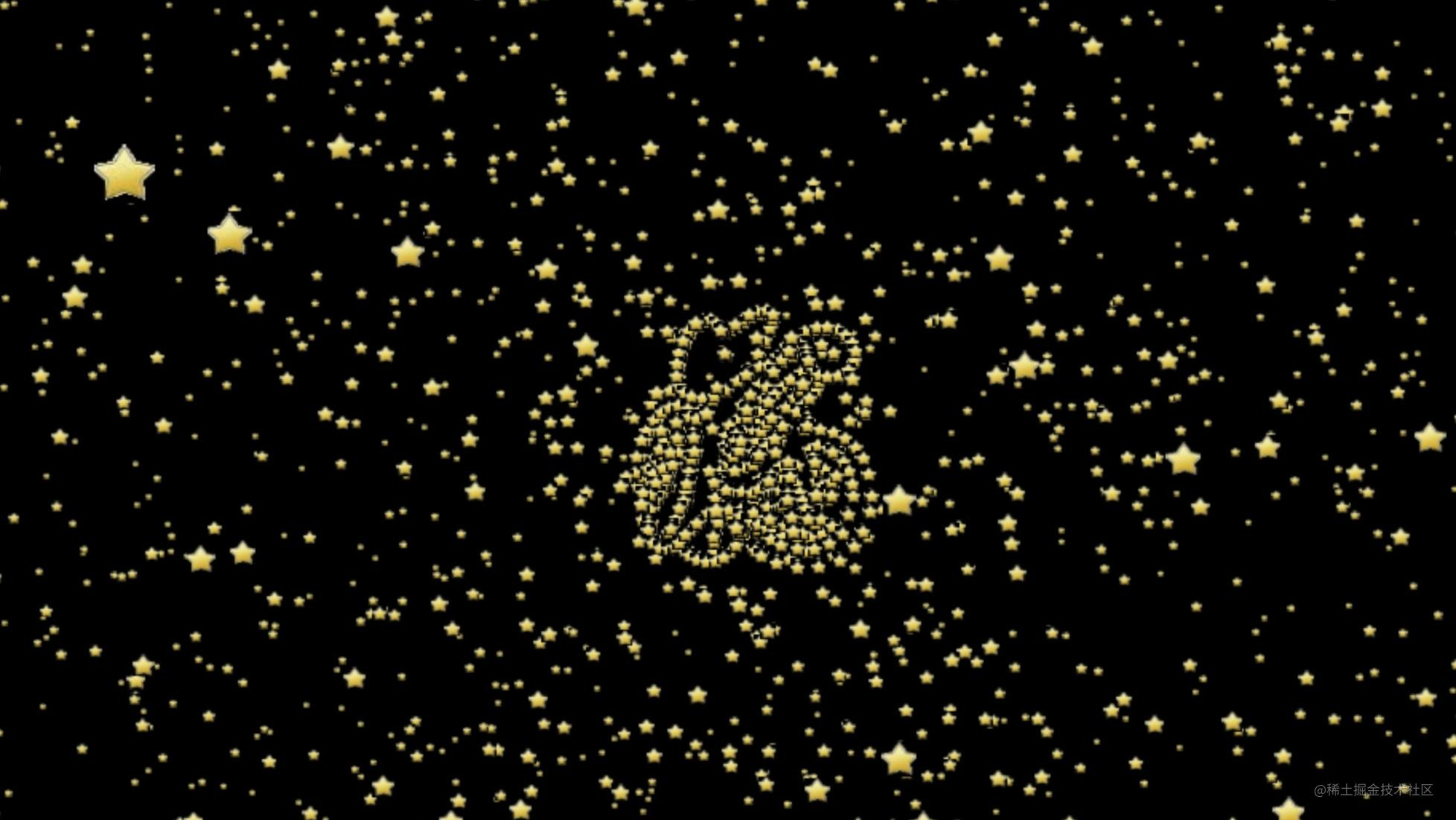PK创意闹新春，我正在参加「春节创意投稿大赛」，详情请看：春节创意投稿大赛

”粒子动画“ 这个词大家可能经常听到，那什么是粒子动画呢？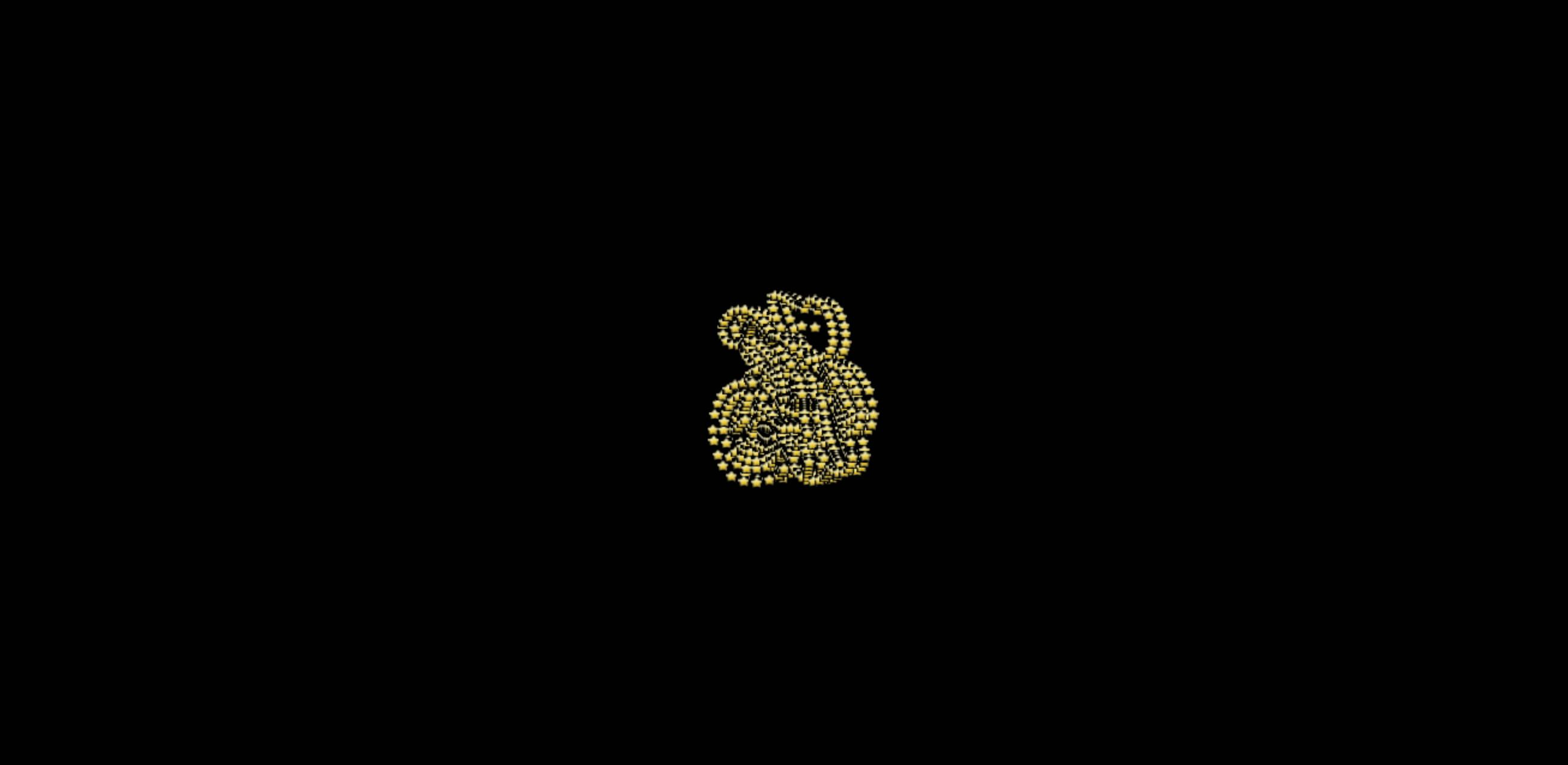## 思路分析

3D 世界中，物体是由顶点构成，3 个顶点构成一个三角形，然后给三角形贴上不同的纹理，这样就是一个三维模型。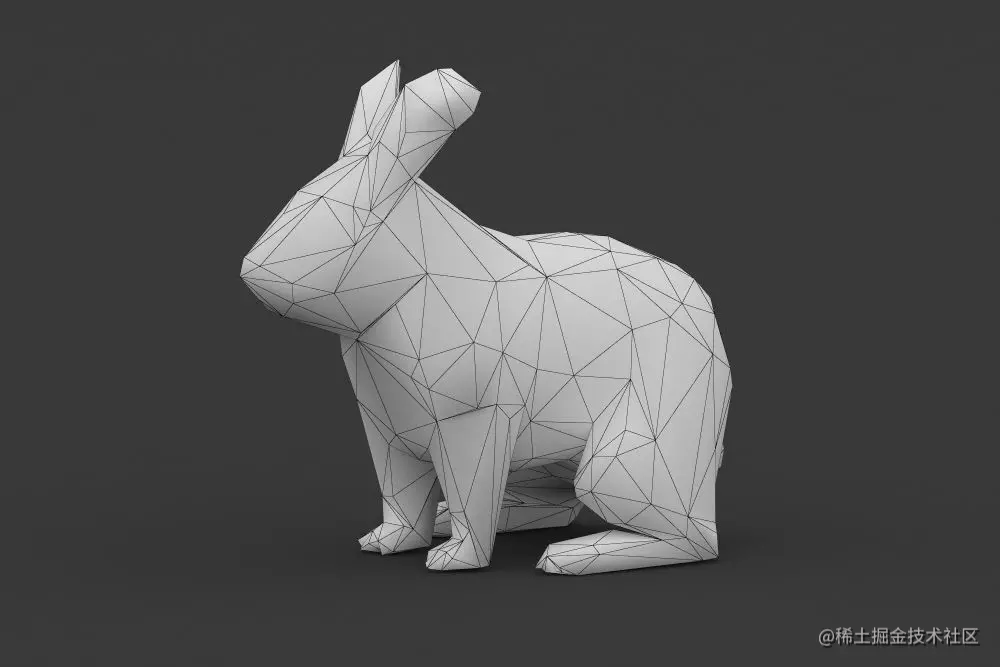3D 物体是由顶点构成，那让这些顶点动起来就是粒子动画了，因为基本粒子动了，自然就会有打碎重组的效果。

## 代码实现

``````const scene = new THREE.Scene();

``````const width = window.innerWidth;
const height = window.innerHeight;
const camera = new THREE.PerspectiveCamera(45, width / height, 0.1, 1000);

``````camera.position.set(100, 0, 400);
camera.lookAt(scene.position);

``````const renderer = new THREE.WebGLRenderer();
renderer.setSize(width, height);
document.body.appendChild(renderer.domElement);

``````function render() {
renderer.render(scene, camera);
requestAnimationFrame(render);
}
render();

### 绘制星空

``````const vertices = [];
for ( let i = 0; i < 30000; i ++ ) {
const x = THREE.MathUtils.randFloatSpread( 2000 );
const y = THREE.MathUtils.randFloatSpread( 2000 );
const z = THREE.MathUtils.randFloatSpread( 2000 );
vertices.push( x, y, z );
}

``````const geometry = new THREE.BufferGeometry();
geometry.setAttribute( 'position', new THREE.Float32BufferAttribute(vertices, 3));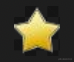``````const star = new THREE.TextureLoader().load('img/star.png');
const material = new THREE.PointsMaterial( { size: 10, map: star });

``````const points = new THREE.Points( geometry, material );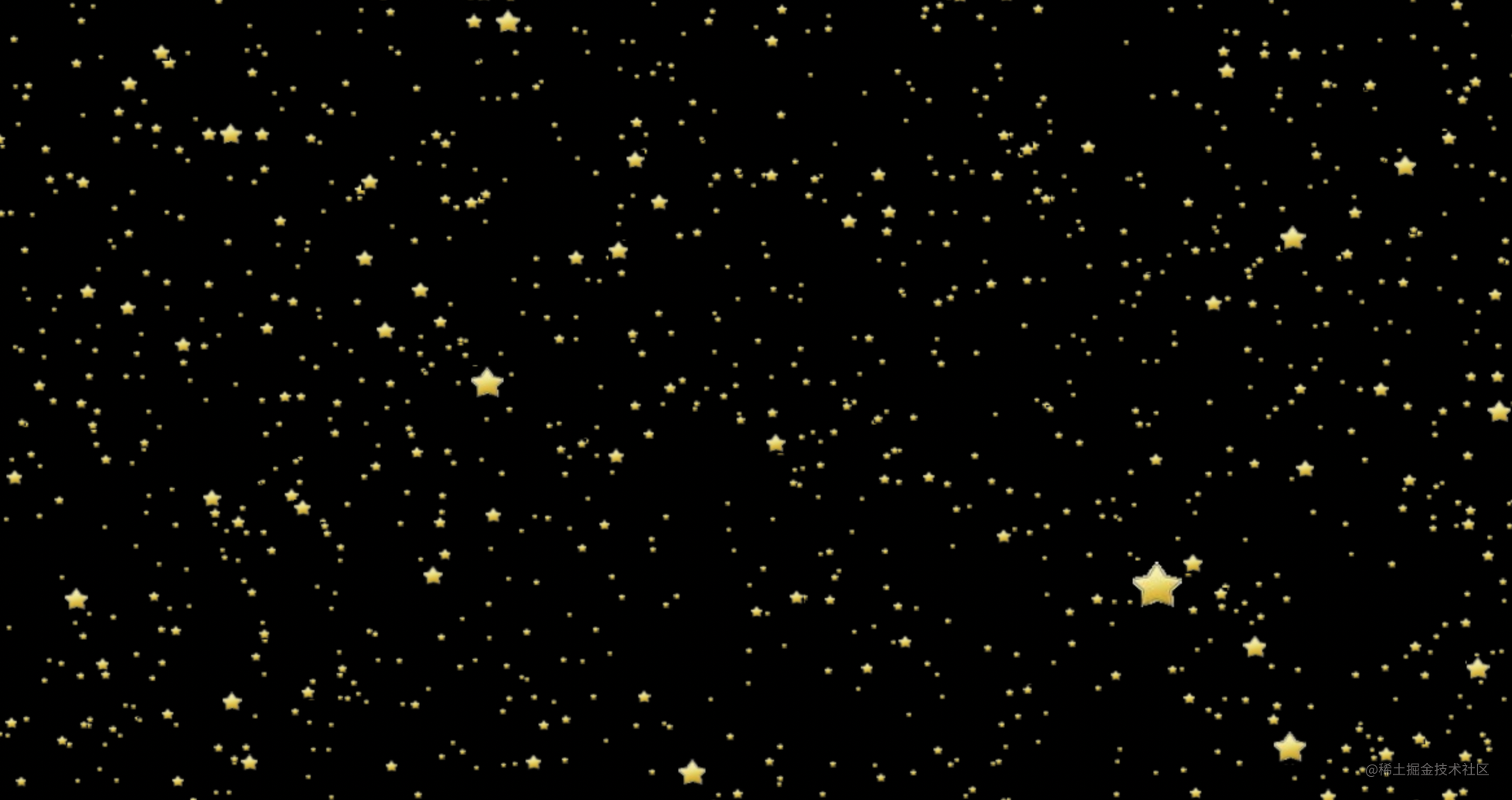``````function render() {
renderer.render(scene, camera);
scene.rotation.y += 0.001;

requestAnimationFrame(render);
}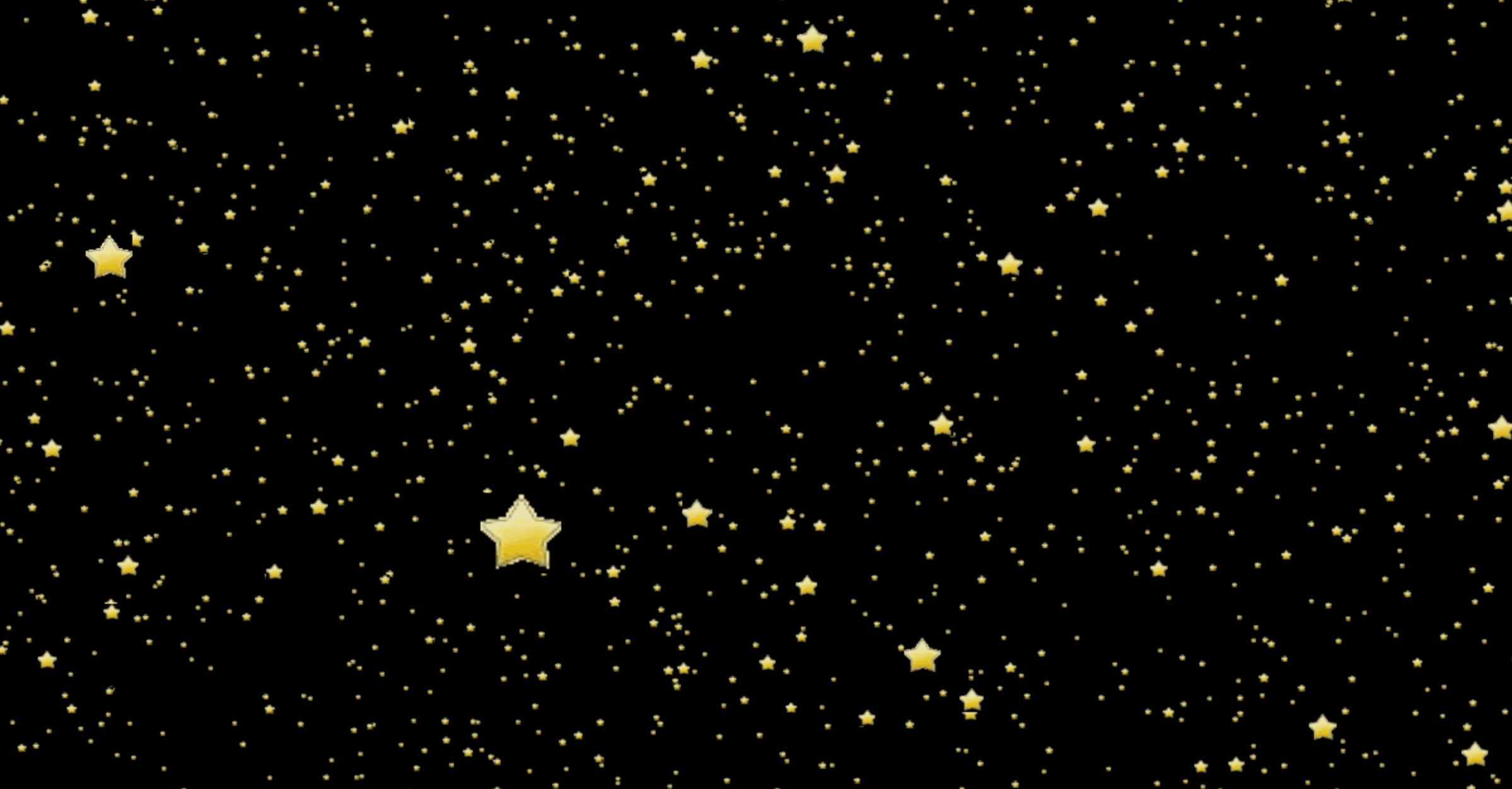3D 星空的感觉有了！

### 3D 粒子动画

3D 粒子动画就是顶点的动画，也就是 x、y、z 的变化。

``````const startPositions = geometry.getAttribute('position');

for(let i = 0; i< startPositions.count; i++) {
const tween = new TWEEN.Tween(positions);

tween.to({
[i * 3]: 0,
[i * 3 + 1]: 0,
[i * 3 + 2]: 0
}, 3000 * Math.random());

tween.easing(TWEEN.Easing.Exponential.In);
tween.delay(3000);
tween.onUpdate(() => {
startPositions.needsUpdate = true;
});

tween.start();
}

``````function render() {
TWEEN.update();
renderer.render(scene, camera);
scene.rotation.y += 0.001;

requestAnimationFrame(render);
}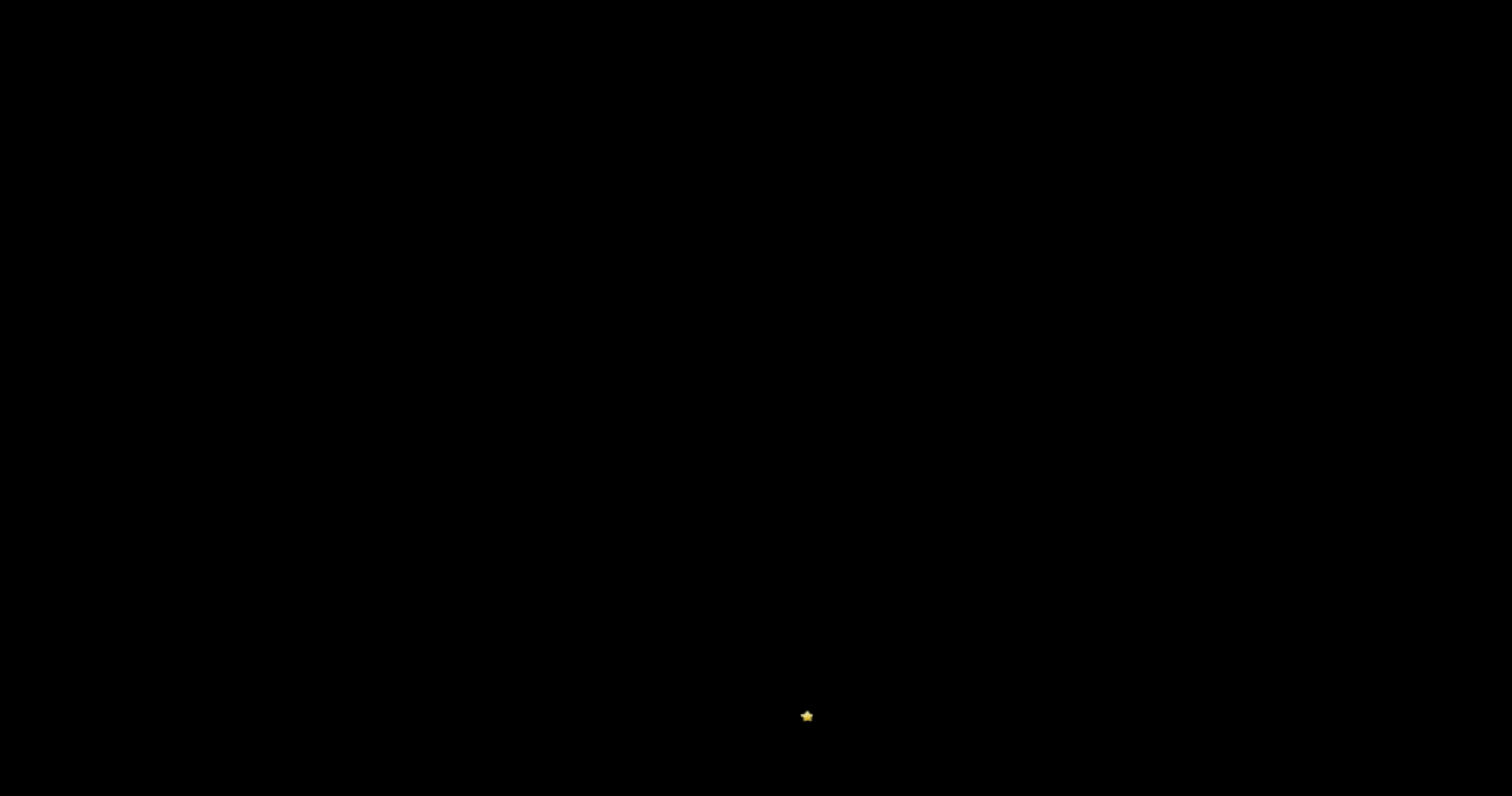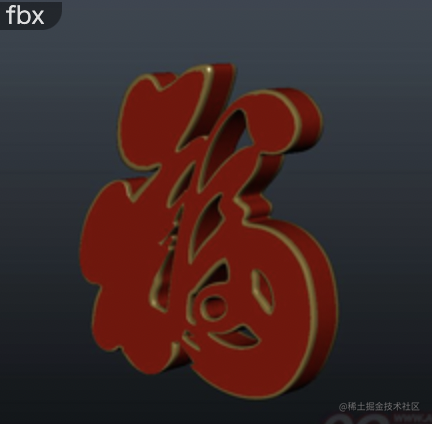``````const loader = new THREE.FBXLoader();
const destPosition = object.children.geometry.getAttribute('position');

});

``````const cur = i % destPosition.count;
tween.to({
[i * 3]: destPosition.array[cur * 3],
[i * 3 + 1]: destPosition.array[(cur * 3 + 1)],
[i * 3 + 2]: destPosition.array[(cur * 3 + 2)]
}, 3000 * Math.random());``````<!DOCTYPE html>
<html lang="en">
<meta charset="UTF-8">
<meta name="viewport" content="width=device-width, initial-scale=1.0">
<title></title>
<style>
body {
margin: 0;
}
</style>
<script src="./js/three.js"></script>
<script src="./js/tween.js"></script>
<script src="./js/TextGeometry.js"></script>
<script src="./js/fflate.js"></script>
<body>
<script>
const width = window.innerWidth;
const height = window.innerHeight;
const camera = new THREE.PerspectiveCamera(45, width / height, 0.1, 1000);

const scene = new THREE.Scene();
const renderer = new THREE.WebGLRenderer();

camera.position.set(100, 0, 400);
camera.lookAt(scene.position);

renderer.setSize(width, height);
document.body.appendChild(renderer.domElement)

function create() {
const vertices = [];
for ( let i = 0; i < 30000; i ++ ) {
const x = THREE.MathUtils.randFloatSpread( 2000 );
const y = THREE.MathUtils.randFloatSpread( 2000 );
const z = THREE.MathUtils.randFloatSpread( 2000 );
vertices.push( x, y, z );
}
const geometry = new THREE.BufferGeometry();
geometry.setAttribute( 'position', new THREE.Float32BufferAttribute( vertices, 3 ) );

const material = new THREE.PointsMaterial( { size: 10, map: star });

const points = new THREE.Points( geometry, material );
points.translateY(-100);

const startPositions = geometry.getAttribute('position');
const destPosition = object.children.geometry.getAttribute('position')
for(let i = 0; i< startPositions.count; i++) {
const tween = new TWEEN.Tween(startPositions.array);
const cur = i % destPosition.count;
tween.to({
[i * 3]: destPosition.array[cur * 3],
[i * 3 + 1]: destPosition.array[cur * 3 + 1],
[i * 3 + 2]: destPosition.array[cur * 3 + 2]
}, 3000 * Math.random());
tween.easing(TWEEN.Easing.Exponential.In);
tween.delay(3000);

tween.start();

tween.onUpdate(() => {
startPositions.needsUpdate = true;
});
}
} );
}

function render() {
TWEEN.update();
renderer.render(scene, camera);
scene.rotation.y += 0.001;

requestAnimationFrame(render);
}
create();
render();
</script>
</body>
</html>